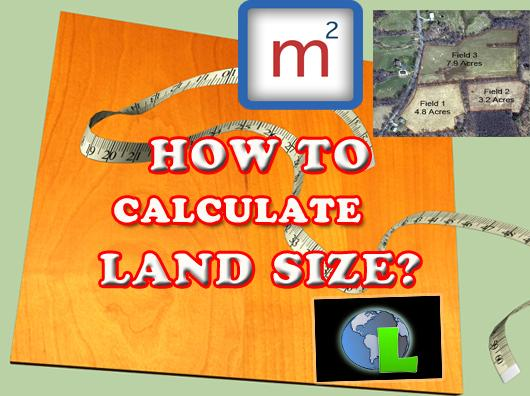# How to Convert Land Size in Hectare, Acre, Point, and Square Feet

If you hadn’t known about how to calculate a land size before buying a land or a house, you might have come across a several property articles and professional sources that collects all these land size calculation information. You want to know all these because you would like to know how big is your land.Chances are if that’s not the case then you may have seen the traditional calculation methods circulating the land and property market. But if you’ve somehow just never came across any of these land size mathematics, you’ll now get to see these formulas showcasing various land area calculation in different systems.

Many of us can’t find the answer regarding 1 Point is equal to how many Square Feet? on the Internet. Hopefully this article can help you. Point system is being used in Sarawak property market because of the land size of Sarawak is big enough. However, the most common system being used in property market in Malaysia is Square Feet and Square Meter.

Here, you will learn how to convert from:
square feet to point
point to square feet
square meter to point
point to square meter
sqft refer to Square Feet = (平方尺)
sqm refer to Square Meter = (平方米)

1 hactare = 2.47105 acre

1 acre = 0.404 hectare

1 sqft = 0.0929 sqm

1 sqm = 10.763 sqft

(LAND SIZE CALCULATION)
1 hectare (公顷) = 10000 sqm
1 acre (英亩) = 4046.856 sqm

1 hectare (公顷) = 107,639 sqft
1 points (点) = 435.5966 sqft

Also,
1 acre = 100 points

100 points has how many Square Feet area?
1 acre = 43559.66 sqft
So, 100 points has 43559.66 sqft of area

100 points has how many Square Meter area?
1 points = 435.5966 sqft
1 acre = 4046.825 sqm
Hence, 100 points has 4046.825 sqm of area

100 points has how many Hectares area?
1 points = 40.46825 sqm
1 acre =0.40469 hectares = 100points

Other calculation information you might want to know:
1 points = 0.0040469 hectares
1 meter = 3.281 feet
1 feet = 0.3048 meter

1 feet = 12 inches
1 cm = 0.3937 inches
1 inches = 2.54 cm = 0.0254 m